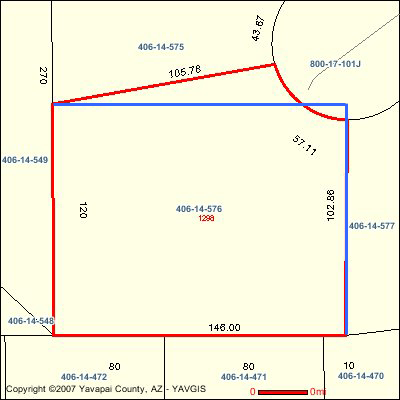SEARCH HOMEMath Central Quandaries & QueriesQuestion from Mimi: I would like to know the square footage of a pentagon with the following measurements: Property is .43 of an Acre Front: 57.11' NW: 105.78' S: 120' SE: 145' E: 102.86' For some reason, I am unable to perform these kinds of equations. I will greatly appreciate your help.Hi Mimi,

We received the drawing you sent but without the angle measurements and more information about the arc at the front of the lot we can't find the area accurately.  I did however find an estimate. Since the angle at the south west corner looks to be approximately a right angle I drew a 120 foot by 146 foot rectangle over your lot with a vertex at this corner.As you can see in the diagram this rectangle has an area slightly smaller that your lot. The area of the rectangle is 120 * 146 = 17520 square feet. There are 43560 square feet in an acre so the area of the rectangle is 17520/43560 = 0.402 acres. The area your county gave you was 0.43 acres so my estimate gives me some confidence that they are correct.

0.43 acres at 43560 square feet per acre is about 18731 square feet.

I hope this helps,
PennyMath Central is supported by the University of Regina and The Pacific Institute for the Mathematical Sciences.